Examples

Chapter 6 Class 12 Application of Derivatives
Serial order wise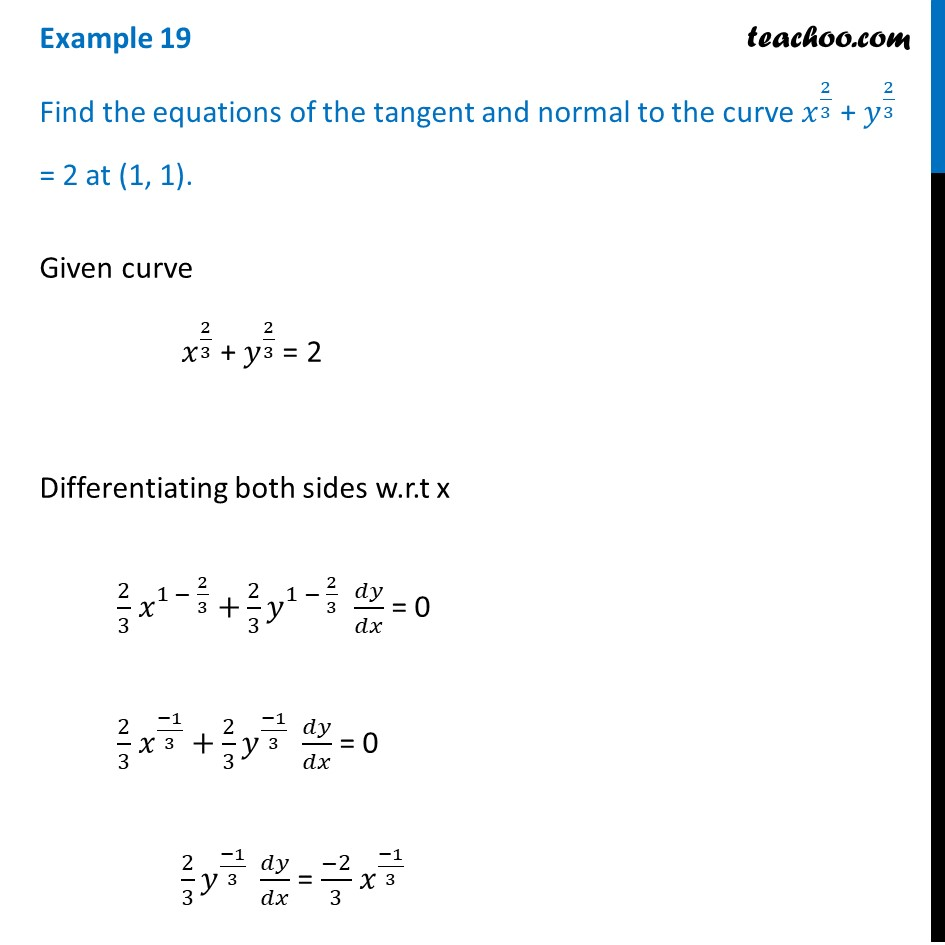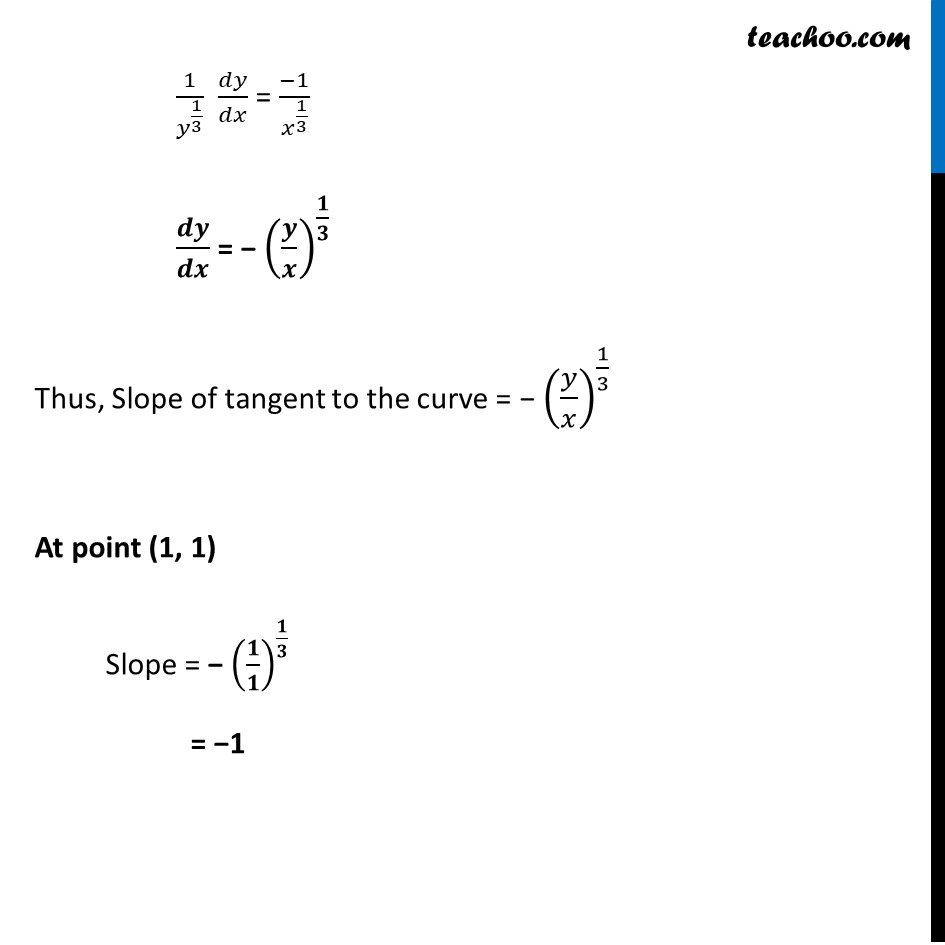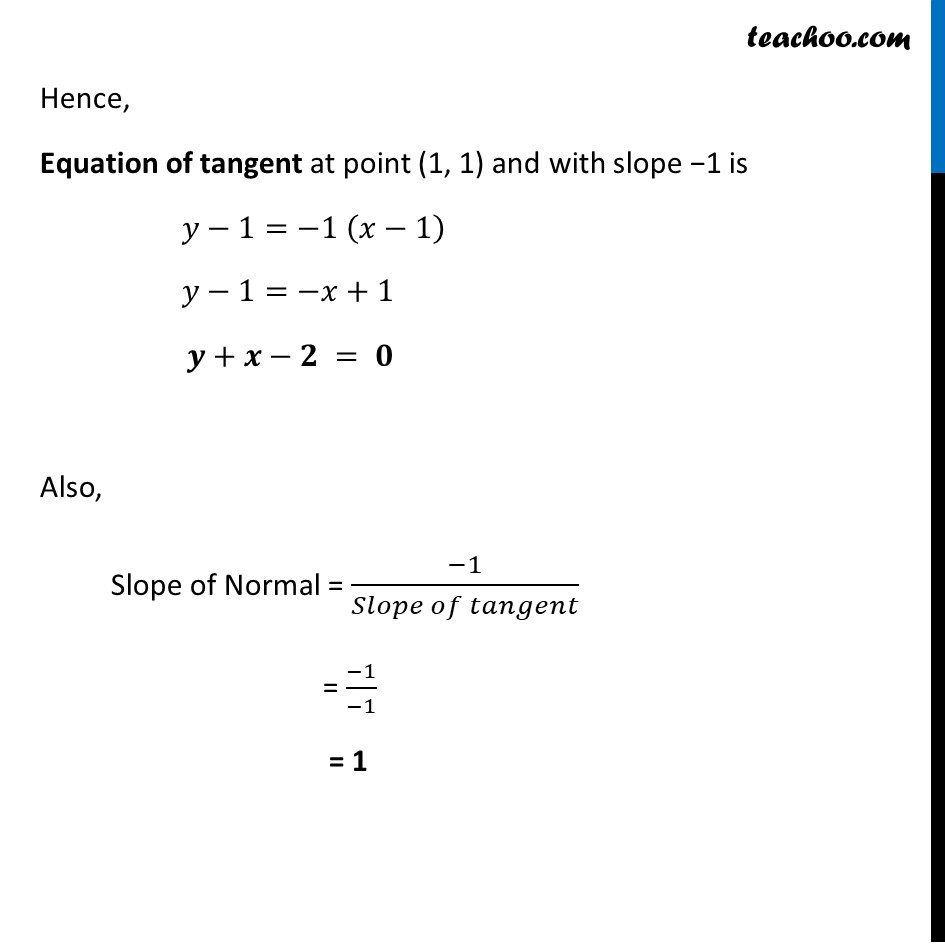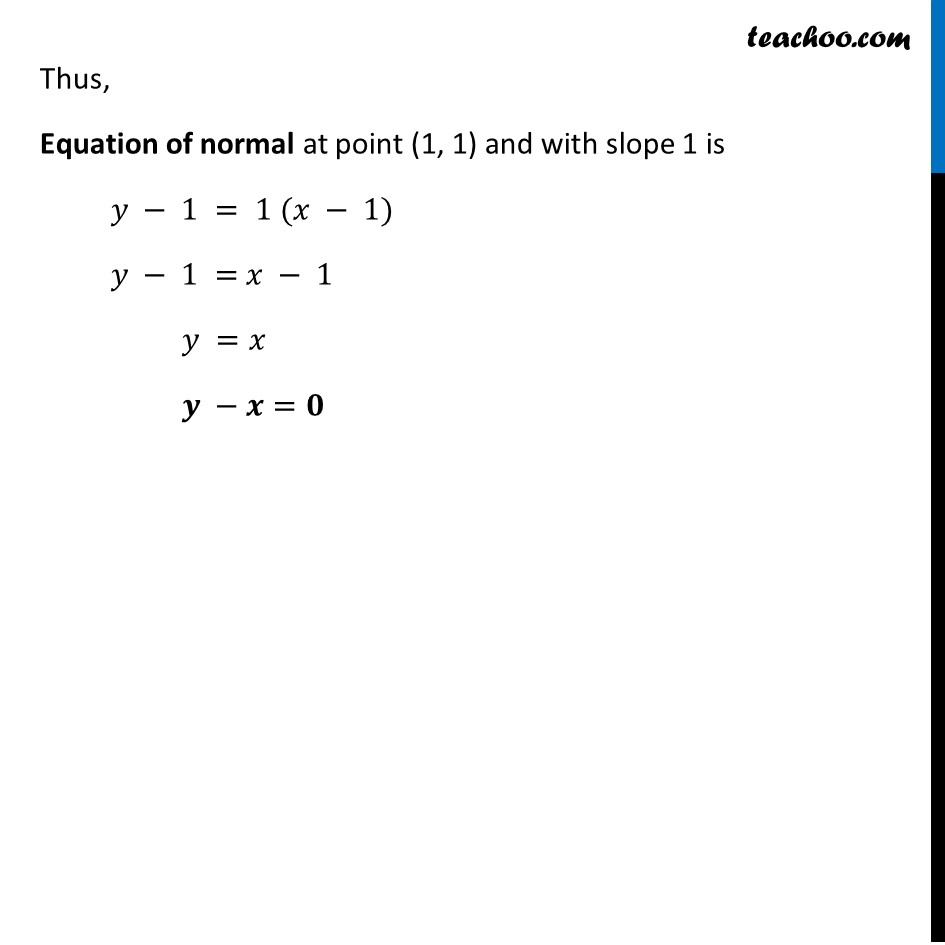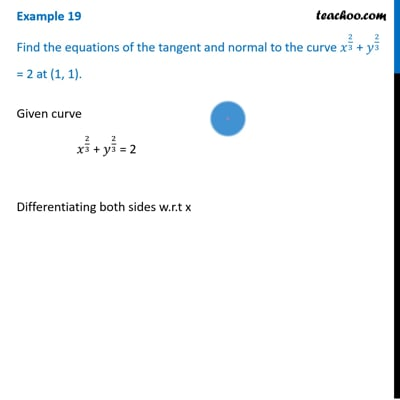This video is only available for Teachoo black users

Introducing your new favourite teacher - Teachoo Black, at only ₹83 per month

### Transcript

Example 19 Find the equations of the tangent and normal to the curve 𝑥^(2/3) + 𝑦^(2/3) = 2 at (1, 1).Given curve 𝑥^(2/3) + 𝑦^(2/3) = 2 Differentiating both sides w.r.t x 2/3 𝑥^(1 − 2/3)+2/3 𝑦^(1 − 2/3) 𝑑𝑦/𝑑𝑥 = 0 2/3 𝑥^((−1)/3)+2/3 𝑦^((−1)/3) 𝑑𝑦/𝑑𝑥 = 0 2/3 𝑦^((−1)/3) 𝑑𝑦/𝑑𝑥 = (−2)/3 𝑥^((−1)/3) 1/𝑦^(1/3) 𝑑𝑦/𝑑𝑥 = (−1)/𝑥^(1/3) 𝒅𝒚/𝒅𝒙 = − (𝒚/𝒙)^(𝟏/𝟑) Thus, Slope of tangent to the curve = − (𝑦/𝑥)^(1/3) At point (1, 1) Slope = − (𝟏/𝟏)^(𝟏/𝟑) = −1 Hence, Equation of tangent at point (1, 1) and with slope −1 is 𝑦−1=−1 (𝑥−1) 𝑦−1=−𝑥+1 𝒚+𝒙−𝟐 = 𝟎 Also, Slope of Normal = (−1)/(𝑆𝑙𝑜𝑝𝑒 𝑜𝑓 𝑡𝑎𝑛𝑔𝑒𝑛𝑡) = (−1)/(−1) = 1 Thus, Equation of normal at point (1, 1) and with slope 1 is 𝑦 − 1 = 1 (𝑥 − 1) 𝑦 − 1 = 𝑥 − 1 𝑦 =𝑥 𝒚 −𝒙=𝟎